AtomicDensityMatrix`
AtomicDensityMatrix`

# Decomposition

Decomposition[op]

returns the expansion coefficients of the decomposition of a rankoperator op (e.g., the density matrix) as a sum of polarization moments.

Decomposition[sys, op]

returns a matrix of expansion coefficients for the decomposition of an operator on an atomic system sys.

# Details and Options

• Decomposition returns either a single tensor decomposition object or a matrix of such objects, each consisting of a list of (contravariant) spherical tensors of increasing rank.
• The decomposition of a rank-operator [i.e., amatrix] is a list of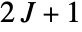spherical tensors of rank zero through.
• Decomposition can also decompose a spherical tensor or Cartesian vector operator, returning a vector or tensor of decompositions.
• The original operator can be recovered using Recomposition.
• TensorForm can be used to display a tensor decomposition as a list of row or column vectors.

# Examples

open allclose all

## Basic Examples(2)

The tensor decomposition of a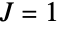operator:

Display the decomposition as a list of column vectors using TensorForm:

Check that it has the form of a contravariant tensor decomposition:

An atomic system consisting of a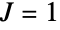state and a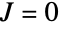state:

Find the matrix of tensor decompositions for the density matrices of the two states and the coherences between the states:

Check that it has the form of a matrix of tensor decompositions:

## Scope(2)

Decomposition of spherical vector operator:

Decomposition of Cartesian vector operator:

## Properties & Relations(3)

The tensor-decomposition components are given by the trace of the product of the operator with the polarization operators.

A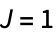operator:

The set of polarization operators for a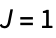space:

The trace of operator times polarization operators:

This is equal to the decomposition of the operator:

The operator can be recovered using Recomposition:

A tensor decomposition can be supplied to AMPSPlot: